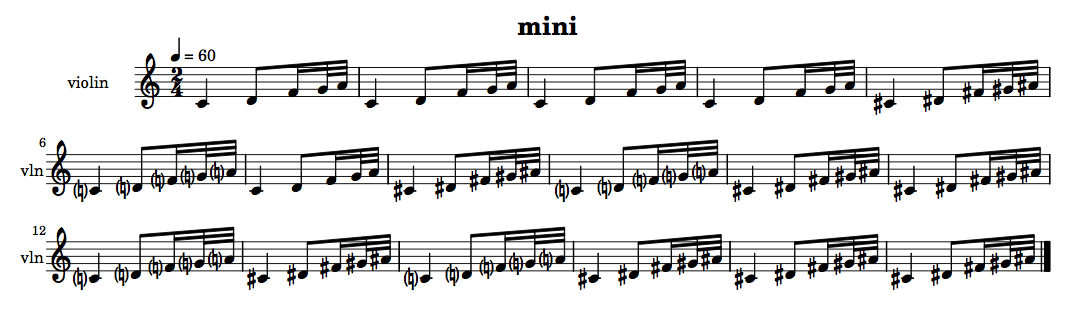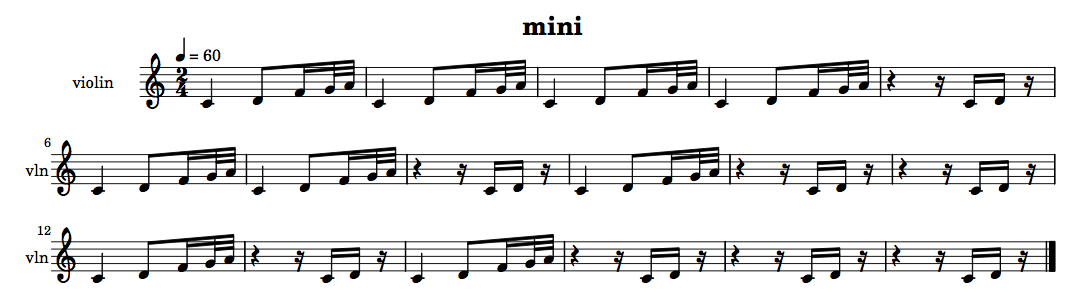## Fibonacci functions

### + Associated example files

NB: An exercise relating to the material covered in this tutorial can be found on the Exercises page.

slippery chicken comes with a number of predefined functions for algorithmic data generation and manipulation, one group of which has its basis in numerical series with Fibonacci relationships. A Fibonacci sequence, named after the 13th-century Italian mathematician Leonardo Fibonacci, is a series of numbers in which each number is the sum of the two preceding numbers in the series, such as (0 1 1 2 3 5 8 13 ...)

### + fibonacci-transition and fibonacci-transitions

#### fibonacci-transition

The slippery chicken function `fibonacci-transition` returns a list in which one repeating element gradually transitions to, and is replaced by, a second repeating element at intervals determined by Fibonacci numbers.

The function first internally determines a list of decreasing Fibonacci numbers, for example (13 8 5 3 2 1). The first element begins repeating and at the 13th repetition is replaced once by the second element. The first element repeats again is then replaced once again at the 8th repetition by the second element, and so on. When the Fibonacci number 1 is reached, the full transition has been completed and the remainder of the new list consists of repetitions of only the second element.

The `fibonacci-transition` function can be called with either one or three arguments. In the first case, it will transition from `0` to `1` over the specified number of total repetitions:

```(fibonacci-transition 50)

=> (0 0 0 0 0 0 0 0 0 0 0 0 1 0 0 0 0 0 0 0 1 0 0 0 0 1 0 0 1 0 1 0 1 0 1 1 0 1
1 1 1 0 1 1 1 1 1 1 1 1)```

If the user would like to transition between two items other than `0` and `1`, this can be done by specifying the two items as the second and third arguments to the function.

```(fibonacci-transition 50 's 'e)

=>
(S S S S S S S S S S S S E S S S S S S S E S S S S E S S E S E S E S E E S E E
E E S E E E E E E E E)```

#### fibonacci-transitions

This same concept can be used to produce a series of consecutive transitions between multiple elements, by using the `fibonacci-transitions` (plural) function. This function also takes as its first argument the number of repetitions over which the entire transition is to occur.

The second argument can either be a single number or a list of items. If the second argument is a single number, the method will apply the `fibonacci-transition` function between each of the sequential numbers starting at `0` and ending at one below the number specified:

```(fibonacci-transitions 76 4)

=> (0 0 0 0 0 0 0 1 0 0 0 0 1 0 0 1 0 1 0 1 1 0 1 0 1 1 1 1 1 1 1 2 1 1 2 1 2 1
2 2 1 2 1 2 2 2 2 2 2 2 3 2 2 3 2 3 2 3 3 2 3 2 3 3 3 2 3 3 3 3 3 3 3 3 3 3)```

If the second argument is a list of items, the function will perform a `fibonacci-transition` from the first to the second element of the list, then from the second to the third and so forth.

```(fibonacci-transitions 304 '(s e q h))

=> (S S S S S S S S S S S S S S S S S S S S S S S S S S S S S E S S S S S S S S
S S S S E S S S S S S S E S S S S E S S S S E S S E S S E S S E S E S E S E
E S E S E S E E S E E S E E E E S E E E E S E E E E E E E E E E E E E E E E
E E E E Q E E E E E E E Q E E E E Q E E E E Q E E Q E E Q E E Q E Q E Q E Q
Q E Q E Q E Q Q E Q Q E Q Q Q Q E Q Q Q Q E Q Q Q Q Q Q Q Q Q Q Q Q Q Q Q Q
Q Q Q Q H Q Q Q Q Q Q Q H Q Q Q Q H Q Q Q Q H Q Q H Q Q H Q Q H Q H Q H Q H
H Q H Q H Q H H Q H H Q H H H H Q H H H H Q H H H H H H H H Q H H H H H H H
H H H H H H H H H H H H H H H H H H H H H H H H H H H H H H H H H H H H H H)```

A detailed example of `fibonacci-transitions` in use can be found in the tutorial to the composition second law.

As of May 2016, both transition functions can take a further optional argument to indicate whether a morph should take place between the items. Instead of a hard switching back and forth, a `morph` structure is returned that indicates that a balanced proportion of the two items should be created. Presently this is only used to do chord/set morphing in a set map. The palette class however has generalised the get-data method to handle morphing of any objects. So as long as a morph method has been defined (e.g. see the chord class) then palettes of any type of data could return a morphed object. See also set-maps.

More detail on these functions can also be found in the source code documentation for `l-for-lookup`.

close

### + The remix-in function

The `remix-in` function is used to modify and lengthen an existing list by inserting consecutive elements from that list back into the list at positions determined by Fibonacci numbers. The method proceeds sequentially through adjacent elements of the original list, and begins mixing earlier elements of the list back into the original list once the third unique element in the original list has been reached.

The earlier elements are mixed back in sequentially starting at the beginning of the original list, and inserted at automatically selected positions within the original list. Thus, if the original list begins with the elements (1 2 3 4 5…), the function will first insert the number 1 back into the original list the at a position automatically selected by Fibonacci numbers, then the number 2 at the next position selected, then the number 3 and so forth.

```(remix-in '(1 2 3 4 5 6 7 8 9 10 11))

=> (1 2 3 4 5 1 6 7 2 8 9 3 10 4 11 5)```

The :remix-in-fib-seed keyword argument determines how often an earlier element is re-inserted into the original list. The lower the number, the more often an earlier element is mixed back in. A value of 1 or 2 will result in each earlier element being inserted after every element of the original (once the third element of the original has been reached).

```(remix-in '(1 2 3 4 5 6 7 8 9 10 11) :remix-in-fib-seed 1)

=> (1 2 3 1 4 2 5 3 6 4 7 5 8 6 9 7 10 8 11 9)```

NB: The affects of this method are less evident on short lists.

A second optional keyword argument for the remix-in function is `:mirror`. When set to `T`, the function will pass backwards through the original list once it has reached the end. This argument defaults to `NIL`.

More detail on these functions can also be found in the source code documentation for `l-for-lookup`.

close

### + Examples of use

The `fibonacci-transition(s)` and `remix-in` functions are not attached to any specific component of the `slippery-chicken` object. They merely create lists, and can be used to generate the data for any object.

For example, this brief piece uses `fibonacci-transition` to gradually progress from one set to the next:

```(let* ((mini
(make-slippery-chicken
'+mini+
:title "mini"
:instrument-palette +slippery-chicken-standard-instrument-palette+
:ensemble '(((vn (violin :midi-channel 1))))
:tempo-map '((1 (q 60)))
:set-palette '((1 ((c4 d4 f4 g4 a4)))
(2 ((cs4 ds4 fs4 gs4 as4))))
:set-map (list (list 1 (fibonacci-transition 17 1 2)))
:rthm-seq-palette '((1 ((((2 4) q - e s 32 32 -))
:pitch-seq-palette ((1 2 3 4 5)))))
:rthm-seq-map '((1 ((vn (1 1 1 1 1 1 1 1 1 1 1 1 1 1 1 1 1))))))))
(midi-play mini :midi-file "/tmp/mini.mid")
(write-lp-data-for-all mini :base-path "/tmp/"))```The next example uses the same call to `fibonacci-transition` to produce its `rthm-seq-map`, resulting in a piece in which the violin gradually transitions from one `rthm-seq` to a second `rthm-seq`.

```(let* ((mini
(make-slippery-chicken
'+mini+
:title "mini"
:instrument-palette +slippery-chicken-standard-instrument-palette+
:ensemble '(((vn (violin :midi-channel 1))))
:tempo-map '((1 (q 60)))
:set-palette '((1 ((c4 d4 f4 g4 a4))))
:set-map '((1 (1 1 1 1 1 1 1 1 1 1 1 1 1 1 1 1 1)))
:rthm-seq-palette '((1 ((((2 4) q - e s 32 32 -))
:pitch-seq-palette ((1 2 3 4 5))))
(2 ((((2 4) (q) (s) - s s - (s)))
:pitch-seq-palette ((1 2)))))
:rthm-seq-map (list (list 1
(list (list 'vn
(fibonacci-transition 17 1 2))))))))
(midi-play mini :midi-file "/tmp/mini.mid")
(write-lp-data-for-all mini :base-path "/tmp/"))```A detailed example of `fibonacci-transitions` in use can be also found in the tutorial to the composition second law.

close